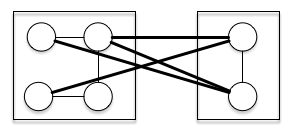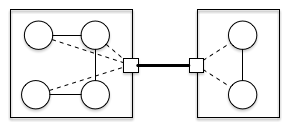与复杂同行（一）7 + 4k < 14 => k < 1.75

3 + 4k < 14 => k < 2.7511+ k < 14 => k < 3

6 + k < 14 => k < 8（待续） 1

1. 前方坑大。管挖不管填。